## Current Issue                           Vol.1. Issue.1.2013.,Research Article A COMMON FIXED POINT THEOREM IN COMPLEX VALUED B-METRIC SPACES K.P.R. RAO*, P.RANGA SWAMY, J.RAJENDRA PRASAD *Department of Mathematics, Acharya Nagarjuna University, Nagarjuna Nagar-522 510, Guntur, (A.P.), India. Abstract In this paper, we give a common fixed point theorem for four maps in complex valued b-metric spaces and obtain a generalization of theorem of R.K.Verma and H.K.Pathak Vol.1. Issue.1.,2013     Page No:1-8                                           Download PDFResearch Article REMARKABLE OBSERVATIONS ON THE HYPERBOLA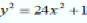M.A.GOPALAN, S.VIDHYALAKSHMI, J.UMARANI* Department of Mathematics, Shrimati Indira Gandhi College, Trichy Abstract The binary quadratic equationis considered and a few interesting properties among the solutions are presented. Employing the integral solutions of the equation under consideration, a few special pythogorean triangles are obtained. Vol.1. Issue.1.,2013     Page No: 9-12                                          Download PDFResearch Article ON HOMOGENEOUS CUBIC EQUATION WITH THREE UNKNOWNS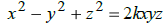M.A.GOPALAN, S. VIDHYALAKSHMI, N.THIRUNIRAISELVI* Department of Mathematics, Shrimati Indira Gandhi College, Tiruchirappalli Abstract The homogeneous cubic equation with three unknowns represented by the diophantine equationis analyzed for its patterns of non – zero integral solutions. A few interesting properties among the solutions are presented. Vol.1. Issue.1.,2013     Page No: 13-15                                          Download PDFResearch Article ON THE HOMOGENEOUS BI-QUADRATIC EQUATION WITH FIVE UNKNOWNS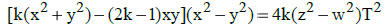M.A.GOPALAN, S. VIDHYALAKSHMI, M.MANJULA* Department of Mathematics, Shrimati Indira Gandhi College,Tiruchirappalli Abstract The biquadratic equation with 5 unknown given byis analyzed for its patterns of non – zero distinct integral solutions. A few interesting relations between the solutions and special polygonal numbers are exhibited. Vol.1. Issue.1.,2013     Page No: 16-21                                        Download PDF   *Page No 22 Intentionally left as blankResearch Article ON THE CUBIC EQUATION WITH EIGHT UNKNOWNS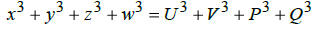M.A.GOPALAN, G.SUMATHI, S.VIDHYALAKSHMI Department of Mathematics, SIGC, Trichy, Abstract We obtain infinitely many non-zero octuple (x, y, z,w,U,V, P,Q) satisfying the cubic equation with eight unknown’s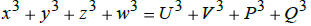. Various interesting relations between the solutions, Polygonal numbers, Pyramidal numbers, and Centered Pyramidal numbers are obtained. Vol.1. Issue.1.,2013     Page No:23-29                                           Download PDFResearch Article A SPECIAL TRANSCENDENTAL EQUATION WITH FIVE UNKNOWNS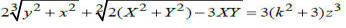S.VIDHYALAKSHMI, K.LAKSHMI*, M.A.GOPALAN Department of Mathematics, Shrimati Indira Gandhi College, Trichy ABSTRACT The transcendental equation with five unknowns represented by the diophantine equationis analyzed for its patterns of non-zero distinct integral solutions and different methods of integral solutions are illustrated. Vol.1. Issue.1.,2013     Page No:30-37                                         Download PDF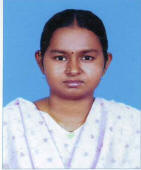Research Article ON THE HOMOGENEOUS QUADRATIC EQUATION WITH THREE UNKNOWNS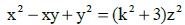M.A.GOPALAN, S. VIDHYALAKSHMI, E.PREMALATHA* Department of Mathematics, Shrimati Indira Gandhi College, Tiruchirappalli Abstract The ternary quadratic Equation with 3 unknown given byis analyzed for its patterns of non – zero distinct integral solutions. A few interesting relations between the solutions and special polygonal numbers are exhibited. Vol.1. Issue.1.,2013     Page No:38-41                              Download PDFResearch Article A NOTE ON ANTI Q-FUZZY KU-SUBALGEBRAS AND HOMOMORPHISM OF KU- ALGEBRAS P.M.SITHAR SELVAM et al Department of Mathematics, PSNA College of Engineering and Technology, Dindigul-624 622, Tamilnadu , India. Abstract In this paper, we introduce the concept of Anti Q-fuzzy KU-subalgebras of KU-algebras. Also we discussed few results of KU-ideal of KU-algebra under homomorphism and anti homomorphism and some of its properties. we proved that if µ and d are anti Q- fuzzy KU- ideals in  KU – algebra X, then µ x d is an anti Q- fuzzy KU-ideal in X x X and few more results in Cartesian product. Vol.1. Issue.1.,2013     Page No:42-49                                  Download PDF Research Article PERFORMANCE OF DISTANCE CLASSIFIER IN PATTERN RECOGNITION NUNNA SRINIVASA RAO Department of Statistics, Andhra Loyola College (Autonomous), Vijayawada ABSTRACT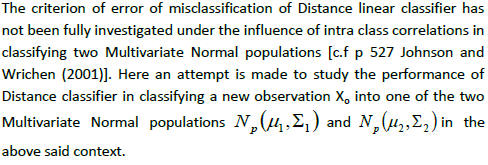. Vol.1. Issue.1.,2013     Page No:50-54                                  Download PDF